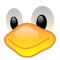(Fabio Alessandro Locati|Fale)'s blog# Sensible integer scale for Gonum Plot

January 20, 2021

Over the years, I found myself multiple times using Gonum Plot. I do find it as a very good and easy to use plotting tool for Go.

The problem I found myself, over and over, dealing with is the tickers scale. If you know before-hand the values that can be expected to be created by the application, it is very straightforward, but the majority of times, this is not the case. I often find myself creating a plotting application on data that track events that have not yet happened and cannot predict their range.

To solve the issue, I create a package that has a struct that implements the Ticker interface and provides tickers that are usually sensible. Since this struct only works for integer scales, I called it `sit`, which stands for “Sensible Int Ticks”.

You can find the `sit` package here, and an example of its usage is:

``````package main

import (
"github.com/fale/sit"
"gonum.org/v1/plot"
"gonum.org/v1/plot/plotter"
"gonum.org/v1/plot/plotutil"
"gonum.org/v1/plot/vg"
)

func main() {
p, err := plot.New()
if err != nil {
return
}

line := plotter.XYs{
plotter.XY{X: float64(1), Y: float64(1)},
plotter.XY{X: float64(2), Y: float64(11)},
plotter.XY{X: float64(3), Y: float64(5)},
plotter.XY{X: float64(4), Y: float64(2)},
plotter.XY{X: float64(5), Y: float64(7)},
}

"First", line,
)
if err != nil {
return
}

p.Y.Tick.Marker = sit.Ticker{}
p.Y.Min = sit.Min(p.Y.Min, p.Y.Max)
p.Y.Max = sit.Max(p.Y.Min, p.Y.Max)

if err := p.Save(10*vg.Inch, 5*vg.Inch, "points.png"); err != nil {
return
}
return
}
``````

The important part is the block between line 33 and 35, that - to be clear - is the following:

``````p.Y.Tick.Marker = sit.Ticker{}
p.Y.Min = sit.Min(p.Y.Min, p.Y.Max)
p.Y.Max = sit.Max(p.Y.Min, p.Y.Max)
``````

The first line of the block ensures that the object that implements the `Ticker` interface from GoNum Plot is used for the Y axes, while the other two make sure that the provided functions from the `sit` package adequately set the min and max Y values.

You can also use it for X ax in a similar way by exchanging `Y` with `X` in the three highlighted lines.

I hope this helps other people as much as it helped me!